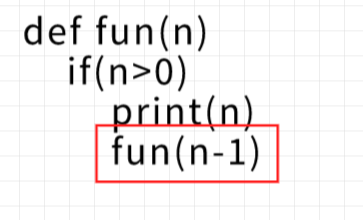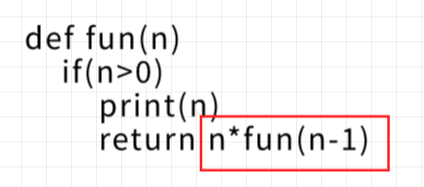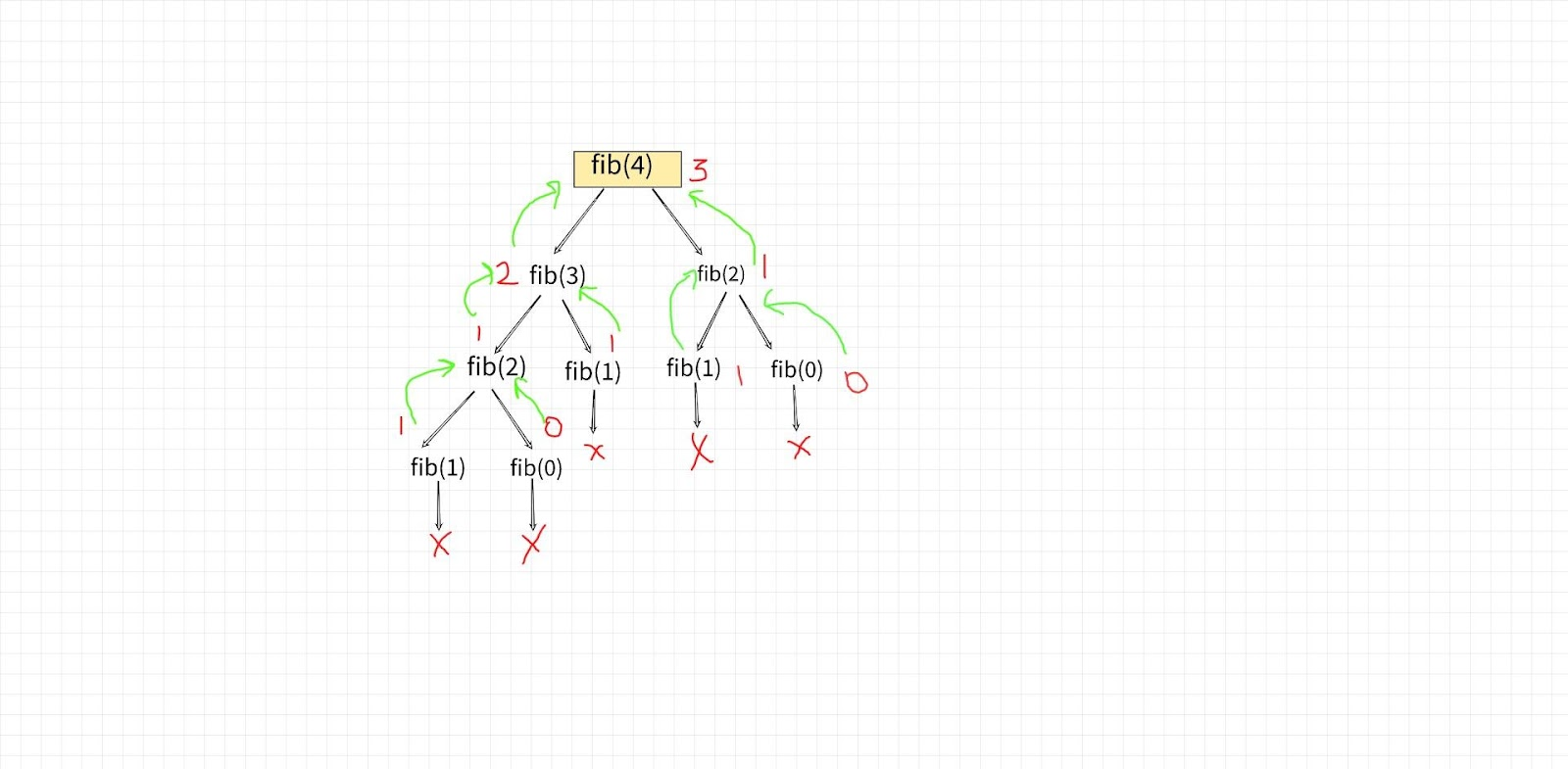# Recursion in Python

Recursion is the process when a function calls itself under some conditions to perform a task repeatedly. The condition under which recursion stops is called Base Case/Condition. The base case tells the function that whenever this condition gets satisfied, stop calling yourself again and again.

• Recursion uses STACK (LIFO) to execute all the calls. First of all, the last recursive call made is executed and then it pops out of the stack space and then the previous call gets executed.
• Recursive functions call their copy under some subproblem with similar arguments. The fundamental idea behind recursion is “Do it for one case, Rest I will do it myself”.

### Basic Structure Of Recursive Functions

```def recur_func( ):

# some code
# to be executed
# base case

recur_func()
```

### Types of Recursion

Types of recursion written in the program depend on the line where the recursive call is made.

If the last statement executed by the function is the recursive call then it is called TAIL Recursion otherwise it is called HEAD Recursion.The call that is highlighted in the pseudocode is the last call that function has made to itself, so this is an example of tail recursion.

There are no more calls to be made but sometimes there is an operation associated with the call and still, some people assume it is a tail recursion which is false. Let us understand it through an example.The statement highlighted is the last statement but there is still a call is associated, the print statement

First waits for the function (n-1) call and then

Multiplies it with n and then return. So, this isn’t an example of tail recursion.

• Direct Recursion: When a function calls itself within the same body.
• Indirect Recursion: When a function (f1) calls another function (f2) which further directly calls f1. This call is called an Indirect recursive call.

Eg: Program to print the Fibonacci series.

Code:

## Python Code

``````def fibonacci(n):
if n <= 1:
return n
else:
return(fibonacci(n-1) + fibonacci(n-2))
n = 5
for i in range(n):
print(fibonacci(i))
``````

Output:

0
1
1
2
3This type of recursion is also called Tree Recursion where there are multiple recursive calls and the recursion tree isn’t a skew tree.

Note: A skew tree is the one that extends in only one direction, either left subtree or right.